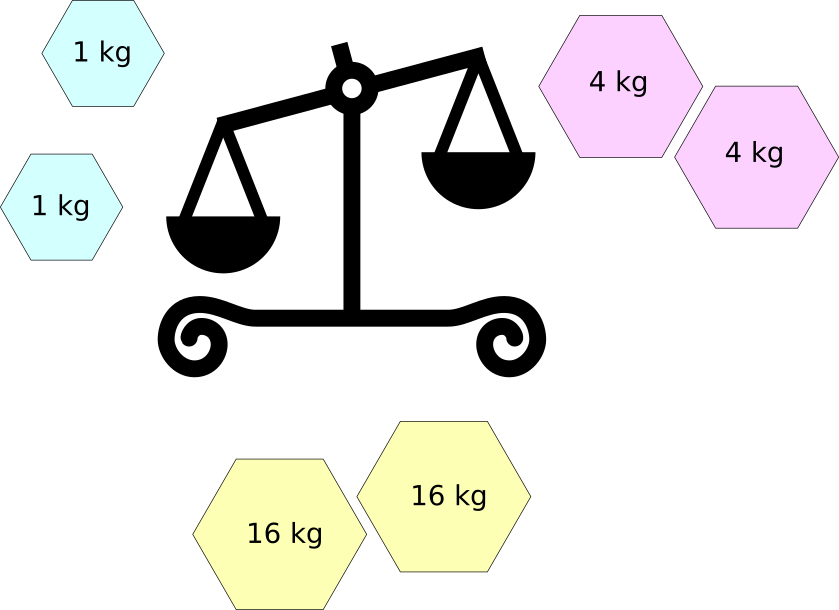# Non-standard Weights

Logic Level 2You have a pan balance, and two weights of $1\text{ kg}, 4\text{ kg}$ and $16\text{ kg}$ each.

How many different positive weights can you measure using just these given weights?

As an explicit example, it is possible to measure 3 kg using these weights. Place a $4\text{ kg}$ weight on one pan, and a $1 \text{ kg}$ weight on the other pan. Only an object weighing 3 kg will be able to balance the pans.

×